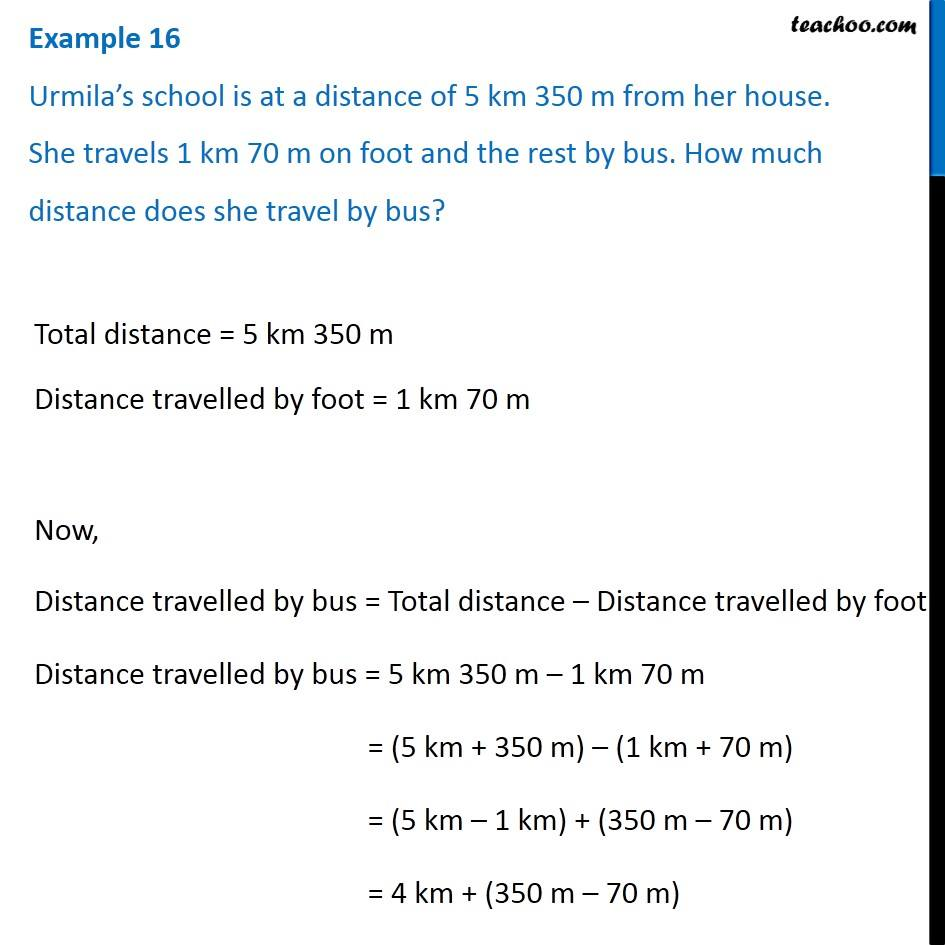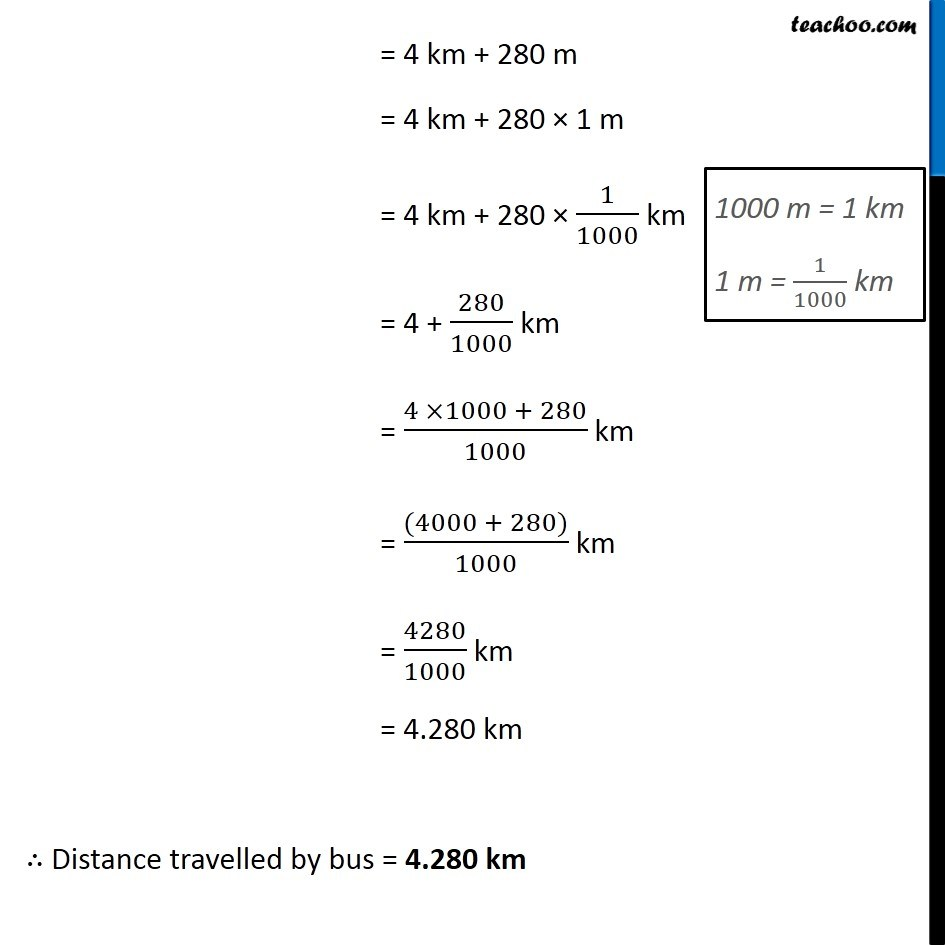Subtraction of decimal numbers - Statement questions

Chapter 8 Class 6 Decimals
Concept wise### Transcript

Example 16 Urmila’s school is at a distance of 5 km 350 m from her house. She travels 1 km 70 m on foot and the rest by bus. How much distance does she travel by bus? Total distance = 5 km 350 m Distance travelled by foot = 1 km 70 m Now, Distance travelled by bus = Total distance – Distance travelled by foot Distance travelled by bus = 5 km 350 m – 1 km 70 m = (5 km + 350 m) – (1 km + 70 m) = (5 km – 1 km) + (350 m – 70 m) = 4 km + (350 m – 70 m) = 4 km + 280 m = 4 km + 280 × 1 m = 4 km + 280 × 1/1000 km = 4 + 280/1000 km = (4 ×1000 + 280)/1000 km = ((4000 + 280))/1000 km = 4280/1000 km = 4.280 km ∴ Distance travelled by bus = 4.280 km